# Swift Algorithm Club: Swift Merge Sort

In this Swift Merge Sort tutorial, you’ll learn how to implement the merge sort algorithm in a step-by-step Playground. By Kelvin Lau.

Leave a rating/review
Save for later
Share

## Swift Algorithm Club: Swift Merge Sort

15 mins

The Swift Algorithm Club is an open source project to implement popular algorithms and data structures in Swift.

Every month, the SAC team will feature a cool data structure or algorithm from the club in a tutorial on this site. If your want to learn more about algorithms and data structures, follow along with us!

In this tutorial, you’ll walk through one of the classics of sorting algorithms, the merge sort.

This algorithm was first implemented by Kelvin Lau, and is now refactored for tutorial format.

Note: New to the Swift Algorithm Club? Check out our getting started post first.

## Getting Started

Invented in 1945 by John von Neumann, merge sort is an efficient sorting algorithm. The idea behind merge sort is to divide and conquer; To break up a big problem into small problems. A helpful mantra to remember merge sort by is split first and merge after.

Assume you’re given a pile of playing cards.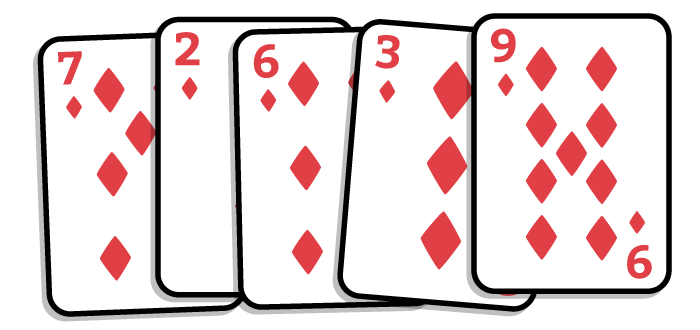The merge sort algorithm works as follows:

1. Split in half. You now have two unsorted piles.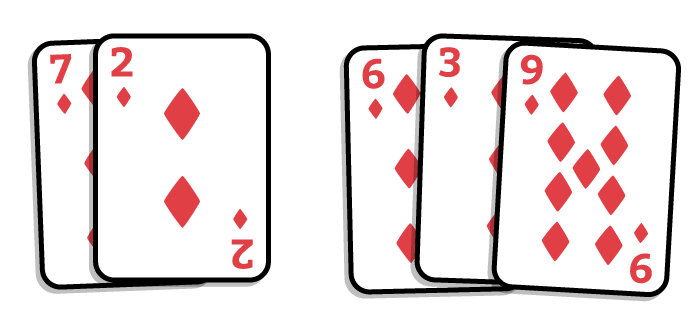2. Keep splitting the resulting piles until you can’t split anymore. In the end, you will have 1 card for each pile.
3. Merge the piles together. During each merge, you put the contents in sorted order. This is easy because each individual pile is already sorted.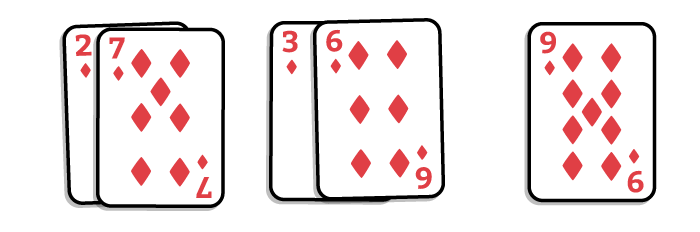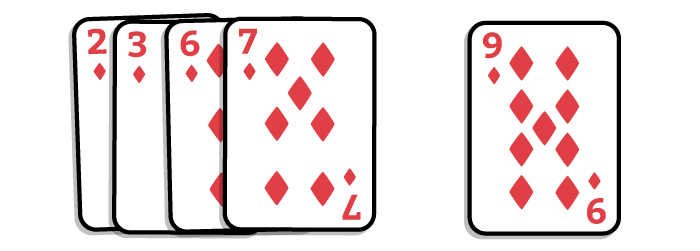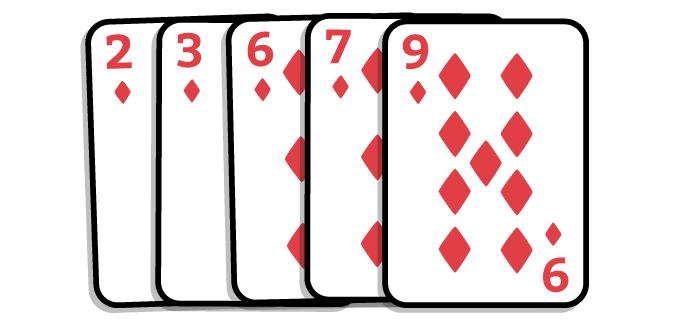## Swift Merge Sort

Let’s see what the merge sort algorithm looks like in Swift.

Open up a new Swift Playground add the following code:

```let array = [7, 2, 6, 3, 9]

func mergeSort(_ array: [Int]) -> [Int] {

}
```

Here you start off with an unsorted array of integers. Your goal is to implement this function that takes an integer array and returns a new array in sorted order.

### 1) Split

Remember your first step is to split the array in half. To do this, update the `mergeSort` function to the following:

```func mergeSort(_ array: [Int]) -> [Int] {
// 1
let middleIndex = array.count / 2
// 2
let leftArray = Array(array[0..<middleIndex])
let rightArray = Array(array[middleIndex..<array.count])
}
```

The code shouldn't compile yet, but don't worry. Your first task is to split the array in two halves.

1. You find the middle index of the array by taking the `count` of the array and dividing it by half.
2. Now that you have the middle index, you create two new arrays. The left array contains the first half of the original array, and the right array contains the second half.

### 2) Keep Splitting

Now that you know how to split the array in half, it's time for the second step: to keep splitting the array until you can't split anymore (i.e. each subdivision contains 1 element). That's a standard use case for recursion.

You will do this using recursion. To do this, update the `mergeSort` function to the following:

```func mergeSort(_ array: [Int]) -> [Int] {
// 1
guard array.count > 1 else { return array }

let middleIndex = array.count / 2

// 2
let leftArray = mergeSort(Array(array[0..<middleIndex]))
let rightArray = mergeSort(Array(array[middleIndex..<array.count]))
}
```

1. All recursive implementations need a base case. You can also think of it as an "exit condition". In your case, you want to stop the recursion when the array only has 1 element.
2. You're now calling `mergeSort` on the left and right halves of the original array. As soon as you've split the array in half, you're going to try to split again.
Note: Understanding recursion can be quite tricky. If you're confused at how this works, try to trace out each step on paper using the test array of `[7, 2, 6, 3, 9]`.

There's still more work to do before your code will compile. Now that you've accomplished the splitting part, it's time to focus on merging.

### 3) Merge

Your final step is to merge the `leftArray` and `rightArray` together. To keep things clean, you will create a separate `merge` function for this.

The sole responsibility of the merging function is to take in 2 sorted arrays and combine them together. The output needs to retain the sorted order. Add the following to the playground:

```func merge(_ left: [Int], _ right: [Int]) -> [Int] {
// 1
var leftIndex = 0
var rightIndex = 0

// 2
var orderedArray: [Int] = []

// merging logic goes here!

return orderedArray
}
```

This is the basic skeleton for the merging function:

1. You've declared 2 variables - `leftIndex` and `rightIndex`. You'll use them to keep track of your progress as you parse through the two arrays.
2. The `orderedArray` will house the combined array.

[spoiler]
Although this implementation is functionally correct, the performance characteristics leaves much to be desired. As mentioned before, the `merge` function is suppose to take in 2 sorted arrays. This is a crucial precondition that allows for optimizations. The `sorted` method doesn't take advantage of this, but you will.
[/spoiler]

Quick quiz:
What's the downside of doing the following?
```
func merge(_ left: [Int], _ right: [Int]) -> [Int] {
let combinedArray = left + right
return combinedArray.sorted(<)
}
```
```
func merge(_ left: [Int], _ right: [Int]) -> [Int] {
let combinedArray = left + right
return combinedArray.sorted(<)
}
```

The strategy is to append elements from the `left` and `right` arrays one by one. Comparisons will be made at each step, ensuring that the smaller of the two elements goes into the `orderedArray`. Update the `merge` function to the following:

```func merge(_ left: [Int], _ right: [Int]) -> [Int] {
var leftIndex = 0
var rightIndex = 0

var orderedArray: [Int] = []

// 1
while leftIndex < left.count && rightIndex < right.count {
// challenge!
}

// 2
while leftIndex < left.count {
orderedArray.append(left[leftIndex])
leftIndex += 1
}

while rightIndex < right.count {
orderedArray.append(right[rightIndex])
rightIndex += 1
}

return orderedArray
}
```

This sets up the things to consider when iterating through the arrays:

1. The idea is to start in the beginning, comparing the elements in `left` and `right` arrays sequentially. If you reached the end of either array, there's nothing else to compare.
2. The first loop guarantees that either `left` or `right` is empty. Since both arrays are sorted, this ascertains that the rest of the contents in the leftover array are equal or greater than the ones currently in `orderedArray`. In this scenario, you'll append the rest of the elements without comparison.Kelvin Lau

Author